Next: Conjugate priors Up: Choice of priors - Previous: Logical and practical role

## Purely subjective assessment of prior probabilities

In principle, the point is simple, at least in one-dimensional problem in which there is good perception of the possible range in which the uncertain variable of interest could lie: try your best to model your prior beliefs. In practice, this advice seems difficult to follow because, even if we have a rough idea of what the value of a quantity should be, the representation of the prior in mathematical terms seems very committal, because a pdf implicitly contains an infinite number of precise probabilistic statements. (Even the uniform distribution says that we believe exactly in the same way to all values. Who believes exactly that?) It is then important to understand that, when expressing priors, what matters is not the precise mathematical formula, but the gross value of the probability mass indicated by the formula, how probabilities are intuitively perceived and how priors influence posteriors. When we say, intuitively, we believe something with a 95% confidence, it means we are almost sure,'' but the precise value (95%, instead of 92% or 98%) is not very relevant. Similarly, when we say that the prior knowledge is modeled by a Gaussian distribution centered around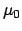with standard deviation[Eq. (28)], it means means that we are quite confident thatis within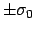, very sure that it is withinand almost certain that it is within3. Values even farther fromare possible, though we do not consider them very likely. But all models should be taken with a grain of salt, remembering that they are often just mathematical conveniences. For example, a textbook-Gaussian prior includes infinite deviations from the expected value and even negative values for physical quantities positively defined, like a temperature or a length. All absurdities, if taken literally. On the other hand, we think that all experienced physicists have in mind priors with low probability long tails in order to accommodate strong deviation from what is expected with highest probability. (Remember that where the prior is zero, the posterior must also be zero.)

Summing up this point, it is important to understand that a prior should tell where the probability mass is concentrated, without taking too seriously the details, especially the tails of the distribution (which should be, however, enough extended to accommodate 'surprises'). The nice feature of Bayes' theorem is the ability of trasform such vague, fuzzy priors into solid estimates, if a sufficient amount of good quality data are at hand. For this reason, the use of improper priors is not considered to be problematic. Indeed, improper priors can just be considered a convenient way of modelling relative beliefs.

In the case we have doubts about the choice of the prior, we can consider a family of functions with some hyperparameters. If we worry about the effect of the chosen prior on the posterior, we can perform a sensitivity analysis, i.e. to repeat the analysis for different, reasonable choices of the prior and check the variation of the result. The final uncertainty could, then, take into account also the uncertainty on the prior. Finally, in extreme cases in which priors play a crucial role and could dramatically change the conclusions, one should refrain to give probabilistic result, providing, instead, only Bayes factors, or even just likelihoods. An example of a recent result about gravitational wave searches presented in this way, see Astone et al (2002).

Having clarified meaning and role of priors, it is rather evident that the practical choice of a prior depends on what is appropriate for the application. For example, in the area of imaging, smoothness of a reconstructed image might be appropriate in some situations. Smoothness may be imposed by a variety of means, for example, by simply setting the logarithm of the prior equal to an integral of the square of the second derivative of the image (von der Linden et al 1996b). A more sophisticated approach goes under the name of Markov random fields (MRF), which can even preserve sharp edges in the estimated images (Bouman and Sauer 1993, Saquib et al 1997). A similar kind of prior is often appropriate for defomable geometric models, which can be used to represent the boundaries between various regions, for example, organs in medical images (Cunningham et al 1998).

A procedure that helps in choosing the prior, expecially important in the cases in which the parameters do not have a straightforwardly perceptible influence on data, is to build a prior predictive pdf and check if this pdf would produce data conform with our prior beliefs. The prior predictive distribution is the analogue of the (posterior) predictive distribution we met in Sect. 5.7, with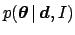replaced by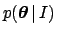(note that the example of Sect. 5.7 was one-dimensional, withand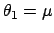), i.e.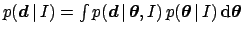.

Often, expecially in complicated data analyses, we are not sufficiently knowledgable about the details of the problem. Thus, informative priors have to be modelled that capture the judgement of experts. For example, Meyer and Booker (2001) show a formal process of prior elicitation which has the aim at reducing, as much as possible, the bias in the experts' estimates of their confidence limits. This approach allows one to combine the results from several experts. In short, we can suggest the use of the coherent bet' (Sect. 2) to force experts to access their values of probability, asking them to provide an interval in which they feel practically sure', intervals on which they could wager 1:1, and so on.Next: Conjugate priors Up: Choice of priors - Previous: Logical and practical role
Giulio D'Agostini 2003-05-13